## Example Questions

### Example Question #1 : How To Solve For A Variable As Part Of A Fraction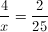Find x.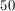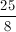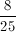None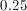Explanation:

Cross multiply: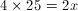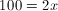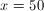### Example Question #1 : How To Solve For A Variable As Part Of A Fraction

The numerator of a fraction is the sum of 4 and 5 times the denominator. If you divide the fraction by 2, the numerator is 3 times the denominator. Find the simplified version of the fraction.Explanation:

Let numerator = N and denominator = D.

According to the first statement,

N = (D x 5) + 4.

According to the second statement, N / 2 = 3 * D.

Let's multiply the second equation by –2 and add itthe first equation:

–N = –6D

+[N = (D x 5) + 4]

=

–6D + (D x 5) + 4 = 0

–1D + 4 = 0

D = 4

Thus, N = 24.

Therefore, N/D = 24/4 = 6.

### Example Question #471 : Algebra

If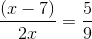, what is the value of?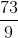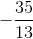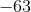Explanation:

Start by cross multiplying to get the equation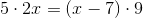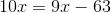.

Then simplify by subtracting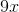from each side to get the solution,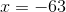.

### Example Question #472 : Algebra

If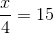, then what is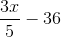equal to?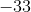Explanation:

We start by solving for x inby multiplying 4 and 15, which gives us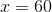.

Then substituting 60 for x in,gives us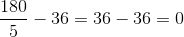which equals 0.

### Example Question #2 : How To Solve For A Variable As Part Of A Fraction

If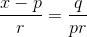, and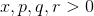, what is the value of?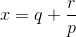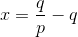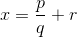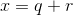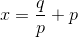Explanation:

Start by cross multiplying which gives us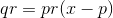.

Simplify by dividing both sides bywhich gives us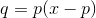.

Isolateby dividing both sides byand then addingto both sides, which gives us.

### Example Question #1 : How To Solve For A Variable As Part Of A Fraction

If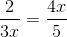, and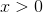what is the value of?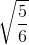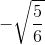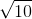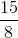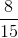Explanation:

Start by cross multiplying which gives us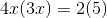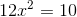.

Divide both sides by 12 and simplify which gives us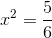.

Since, we only take the positive square root as the solution,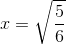.

### Example Question #4 : How To Solve For A Variable As Part Of A Fraction

If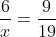, then what is the value of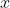?

9/114

3/38

7/12

none of these

38/3

38/3

Explanation:

cross multiply:

(6)(19) = 9x

114=9x

x = 38/3

Tired of practice problems?

Try live online GRE prep today.